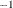# The SIMLIN Procedure

### OUTEST= Data Set

The OUTEST= data set contains all the variables read from the EST= data set. The variables in the OUTEST= data set are as follows.

• the BY statement variables, if any

• _TYPE_, a character variable that identifies the type of observation

• _DEPVAR_, a character variable containing the name of the dependent variable for the observation

• the endogenous variables

• the lagged endogenous variables

• the exogenous variables

• INTERCEPT, a numeric variable containing the intercept values

• _MODEL_, a character variable containing the name of the equation

• _SIGMA_, a numeric variable containing the estimated error variance of the equation (output only if present in the EST= data set)

The observations read from the EST= data set that supply the structural coefficients are copied to the OUTEST= data set, except that the signs of endogenous coefficients are reversed. For these observations, the _TYPE_ variable values are the same as in the EST= data set.

In addition, the OUTEST= data set contains observations with the following _TYPE_ values:

REDUCED

the reduced form coefficients. The endogenous variables for this group of observations contain the inverse of the endogenous coefficient matrix G. The lagged endogenous variables contain the matrix=GC. The exogenous variables contain the matrix=GB.

IMULTi

the interim multipliers, if the INTERIM= option is specified. There are gn observations for the interim multipliers, where g is the number of endogenous variables and n is the value of the INTERIM=n option. For these observations the _TYPE_ variable has the value IMULTi, where the interim number i ranges from 1 to n.

The exogenous variables in groups of g observations that have a _TYPE_ value of IMULTi contain the matrix Dof multipliers at interim i. The endogenous and lagged endogenous variables for this group of observations are set to missing.

TOTAL

the total multipliers, if the TOTAL option is specified. The exogenous variables in this group of observations contain the matrix (I-D ). The endogenous and lagged endogenous variables for this group of observations are set to missing.